## Functions, Graphs and Statistics Test 2

Instructions

DIRECTIONS for the following questions: These questions are based on the situation given below: In each of the questions a pair of graphs F(x) and F1(x) is given. These are composed of straight-line segments, shown as solid lines, in the domain $$x\epsilon (-2, 2)$$.

a. If F1(x) = - F(x)

b. if F1(x) = F(- x)

c. if F1(x) = - F(- x)

d. if none of the above is true

Q 1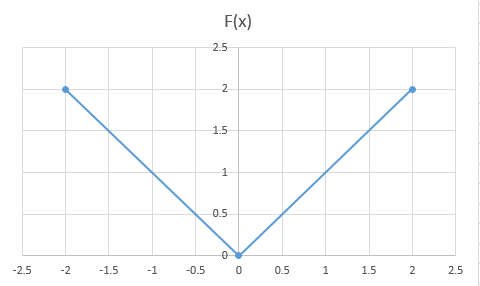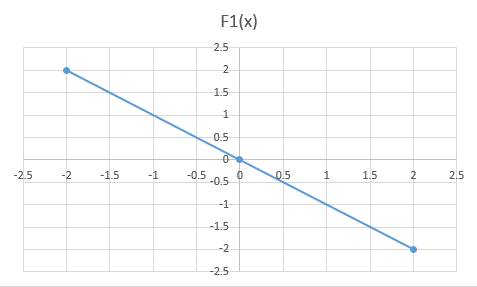Q 2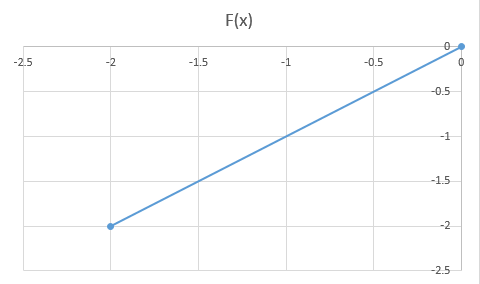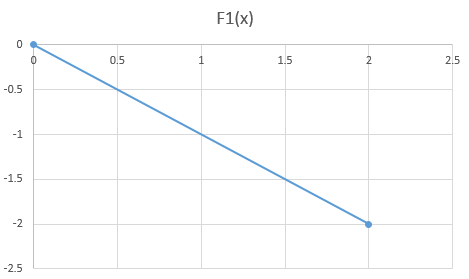Q 3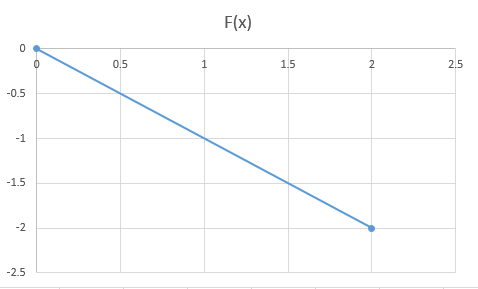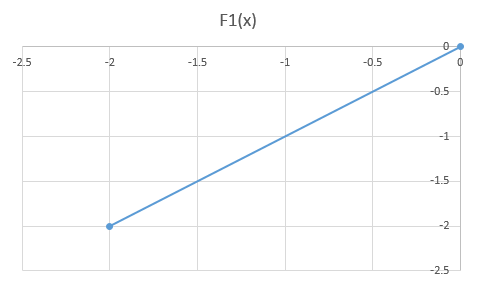Q 4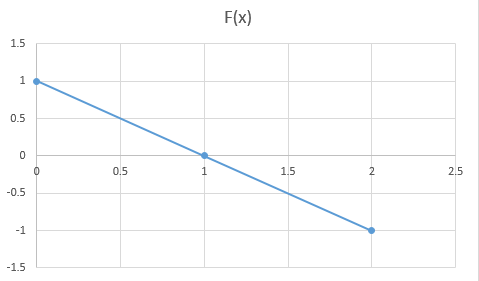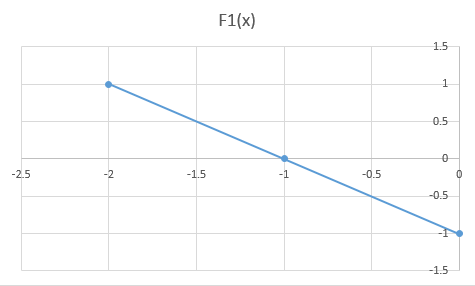Instructions

For the following questions answer them individually

Q 5

Find the maximum value of the expression $$\frac{a^k}{1+a+a^2+a^3.....+a^{2k}}$$ provided a>0 and k is a positive integer.